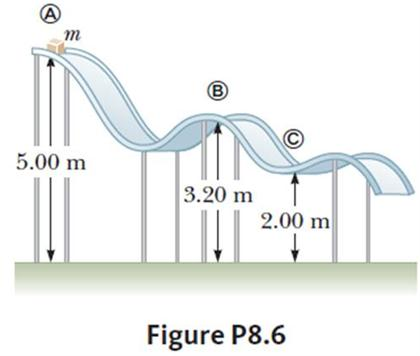Energy is the simplestA block of mass m= 5kg is released from point (a) and slides on the frictionless track shown in the figure. What is the sum of the block's speed at points (b) and (c)

Vb+Vc = ?

Hint: use the mechanical change in energy formula to solve this problem

×